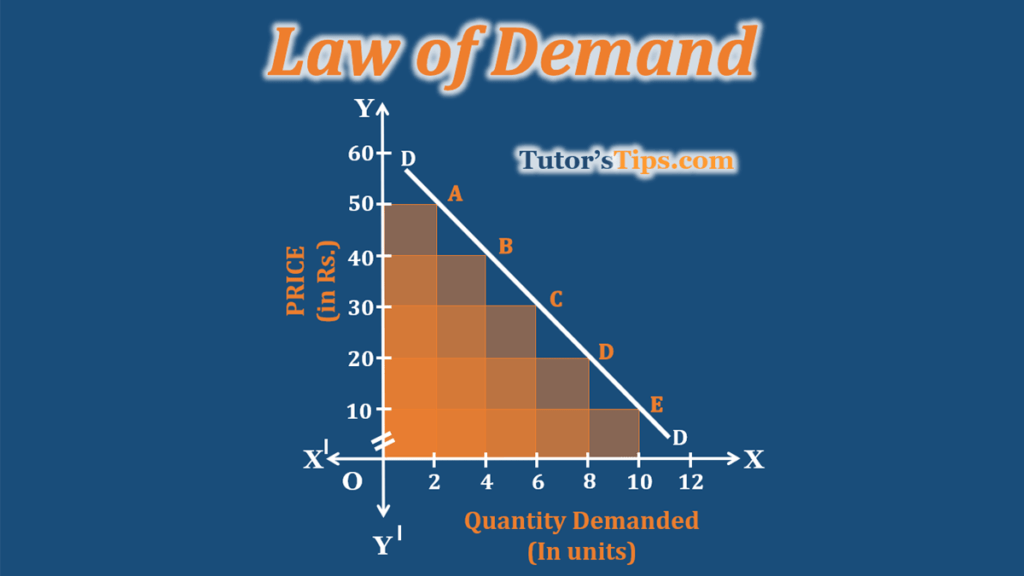# Law of Demand – Explained with ExampleLaw of demand expresses the relationship between price and quantity demanded of a given commodity. It states that “ the quantity demanded increases with a fall in price and diminishes with rising in price, other things being equal.” This happens because of the law of diminishing marginal utility.

Thus, it shows the inverse relationship between quantity demanded of a given commodity and its price. This law defines the direction in which quantity demanded changes with a change in price. The ‘ other things’ includes all those factors which influence the demand such as the income of consumer, price of related goods, tastes of consumer and fashion etc.

The following topics are cover under the chapter of Law of Demand:

## Definition of the Law of Demand:

According to Prof. Marshall, “ The law of demand states that amount demanded increases with fall in price and diminishes when price increases.”

According to Benham, “ Usually a larger quantity of commodity will demand at a lower price than a higher price.”

According to Ferguson, “According to the law of demand, the quantity demanded varies inversely with price.”

## Assumptions of the Law of Demand:

1. There is no change in the tastes and preferences of consumers in a given time period.
2. The income of consumer remains constant for that period of time.
3. There is no change in the price of related goods.
4. There is no change in the quality of that commodity.
5. The habits of consumers remain unchanged during that period of that time.
6. There are no expectations regarding the change in price by consumers.
7. The given commodity is a normal good and has no substitute.

## Characteristics of the law of demand :

1. There is an inverse relationship between price and quantity demanded.
2. Price is the independent variable.
3. Demand is the dependent variable on the price of that commodity.

For example, when the price of 1 kg of mangoes goes down from Rs.80 to Rs. 50, the quantity demanded will go up. Many people who were not able to buy at Rs.80, are now able to purchase at Rs 50.

Similarly, if local Starbucks raises the price of coffee from Rs 700 to Rs. 1000, the quantity demanded will be decreased. Fewer people will buy their coffee, rather they prefer to make their own at home because of the increased price.

## Illustration of the law of demand:

The law of demand can be illustrated with the help of the demand schedule and demand curve. These are shown as follows: –

### Demand schedule:

The following schedule shows the series of prices and the quantity demanded ice cream respectively:

 PRICE OF ICE CREAM QUANTITY DEMANDED 50 2 40 4 30 6 20 8 10 10

The above table shows that when the price of Rs 50, there were 2 units of ice cream demanded. As the price goes down to Rs 40, the quantity demanded increases to 4 units. Similarly, the decline in price to Rs.30, 20, 10 follows an increase in quantity demanded as 6, 8 and 10 units respectively.

### Demand Curve:

The following graph shows the relationship between price and quantity demanded as Demand of Law expresses. In the graph, X-axis shows the quantity demanded of ice cream and Y-axis shows the price. DD is the demand curve whereas the points A, B, C, D and E show the relationship between price and quantity demanded. When the price is Rs.50, the quantity demanded ice cream is 2 units. As price decreases to Rs.40, the quantity demanded increases to 4 units. Similarly, as the price reduces to Rs 30, 20 and 10, the quantity demanded ice cream increases to 6, 8 and 10 units respectively.

Check out Business Economics Books@ Amazon.in